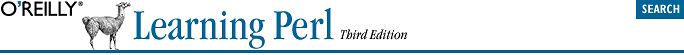home | O'Reilly's CD bookshelfs | FreeBSD | Linux | Cisco | Cisco ExamChapter 15. Strings and Sorting

As we mentioned near the beginning of this book, Perl is designed to be good at solving programming problems that are about 90% working with text and 10% everything else. So it's no surprise that Perl has strong text processing abilities, including all that we've done with regular expressions. But sometimes the regular expression engine is too fancy, and you'll need a simpler way of working with a string, as we'll see in this chapter.

15.1. Finding a Substring with index

Finding a substring depends on where you have lost it. If you happen to have lost it within a bigger string, you're in luck, because the index function can help you out. Here's how it looks:

\$where = index(\$big, \$small);

Perl locates the first occurrence of the small string within the big string, returning an integer location of the first character. The character position returned is a zero-based value -- if the substring is found at the very beginning of the string, index returns 0. If it's one character later, the return value is 1, and so on. If the substring can't be found at all, the return value is -1 to indicate that. In this example, \$where gets 6:

Former C programmers will recognize this as being like C's index function. Current C programmers ought to recognize it as well -- but by this point in the book, you should really be a former C programmer.

my \$stuff = "Howdy world!";
my \$where = index(\$stuff, "wor");

Another way you could think of the position number is the number of characters to skip over before getting to the substring. Since \$where is 6, we know that we have to skip over the first six characters of \$stuff before we find wor.

The index function will always report the location of the first found occurrence of the substring. But you can tell it to start searching at a later point than the start of the string by using the optional third parameter, which tells index to start at that position:

my \$stuff  = "Howdy world!";
my \$where1 = index(\$stuff, "w");               # \$where1 gets 2
my \$where2 = index(\$stuff, "w", \$where1 + 1);  # \$where2 gets 6
my \$where3 = index(\$stuff, "w", \$where2 + 1);  # \$where3 gets -1 (not found)

(Of course, you wouldn't normally search repeatedly for a substring without using a loop.) That third parameter is effectively giving a minimum value for the return value; if the substring can't be found at that position or later, the return value will be -1.

Once in a while, you might prefer to have the last found occurrence of the substring. You can get that with the rindex function. In this example, we can find the last slash, which turns out to be at position 4 in a string:

Well, it's not really the last one found -- Perl cleverly starts searching from the other end of the string, and then returns the first location it finds, which amounts to the same result. Of course, the return value is the same zero-based number as we always use for describing locations of substrings.

my \$last_slash = rindex("/etc/passwd", "/");  # value is 4

The rindex function also has an optional third parameter, but in this case it effectively gives the maximum permitted return value:

my \$fred = "Yabba dabba doo!";
my \$where1 = rindex(\$fred, "abba");  # \$where1 gets 7
my \$where2 = rindex(\$fred, "abba", \$where1 - 1);  # \$where2 gets 1
my \$where3 = rindex(\$fred, "abba", \$where2 - 1);  # \$where3 gets -1